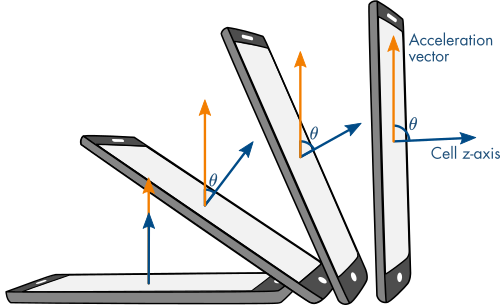## Vector Arithmetic

version 1.0.0 (14.9 MB) by
Interactive courseware module that addresses the fundamentals of vectors taught in mathematics courses.

Updated 21 Jun 2021

From GitHub

# Vector ArithmeticCurriculum Module
Created with R2020b. Compatible with R2020b and later releases.## Description

This curriculum module contains interactive live scripts that teach the fundamental concepts of vectors, such as vector magnitude and addition. These methods are motivated by an application: calculating the orientation of a cell phone using the built-in accelerometer and magnetometer. Throughout the module, students apply the mathematical techniques to computing meaningful values, such as pitch and yaw. These lessons can be used as part of a lecture, as activities in an instructional setting, or as an interactive assignment to be completed outside of class.

The instructions inside the live scripts will guide you through the exercises and activities. Get started with each live script by running it one section at a time. To stop running the script or a section midway (for example, when an animation is in progress), use the Stop button in the RUN section of the Live Editor tab in the MATLAB Toolstrip.

## Details

`vectorBasics.mlx, vectorBasicsSoln.mlx`
An interactive lesson that teaches the basics of individual vectors, such as vector components, magnitude, and orientation. These concepts are applied to compute the pitch of a cell phone using accelerometer readings.

Learning Goals

• Relate the components of a vector to its visualization in 2- and 3-dimensions
• Compute the magnitude of a vector in 2- and 3-dimensions
• Compute the orientation of a vector in 2-dimensions
• Discuss the meaning of the magnitude of an accelerometer reading
• Relate the pitch of a cell phone to accelerometer readings

`vectorArithmetic.mlx, vectorArithmeticSoln.mlx`
An interactive lesson that teaches vector arithmetic. Vector addition, scalar multiplication, dot product, and cross product are discussed. These concepts are applied to compute the yaw of a cell phone using magnetometer readings.

Learning Goals

• Compute scalar, dot, and cross products of vectors
• Relate arithmetic vector operations to visual representations
• Identify the physical meaning of the results of arithmetic vector operations
• Apply vector arithmetic to compute the yaw of a cell phone

`accelerometerReadings.mp4`
An example of the accelerometer readings used in `vectorBasics.mlx`.

` magnetometerReadings.mp4`
An example of the accelerometer and magnetometer readings used in `vectorArithmetic.mlx`.

## Products

MATLAB®, Symbolic Math Toolbox™

The license for this module is available in the LICENSE.TXT file in this GitHub repository.

## Educator Resources

Have any questions or feedback? Contact the MathWorks online teaching team.

### Cite As

Brian Hong (2021). Vector Arithmetic (https://github.com/MathWorks-Teaching-Resources/Vector-Arithmetic/releases/tag/v1.0.0), GitHub. Retrieved .

##### MATLAB Release Compatibility
Created with R2020b
Compatible with R2020b and later releases
##### Platform Compatibility
Windows macOS Linux